Study Guide

# The Fundamental Theorem of Calculus - Units

## Units

Velocity is the rate of change of distance, and is measured in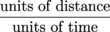When we integrate velocity over a specific time interval, we get the distance traveled over that interval.

The definite integral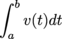can also be defined as the limit, as n goes to ∞, of the left-hand sums LHS(n) for v(t) on [a, b]. To find a left-hand sum we add up the areas of a bunch of rectangles. Check out one of those rectangles. The general case is on the left; a specific example is on the right.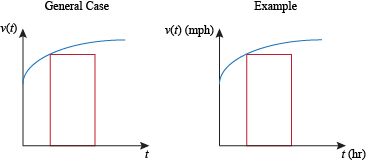The height of the rectangle is measured in the same units as the velocity function, that is,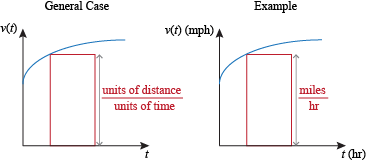The width of the rectangle is measured in the same units as x, that is, units of time: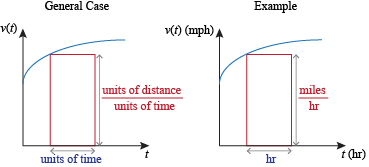We find the area of the rectangle by multiplying its height and its width:

area = height × width.

Similarly, we find the units of the rectangle's area by multiplying the units of its height and the units of its width:

units of area = (units of height)(units of width).

In the case of the velocity function, this means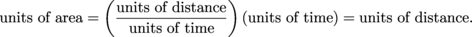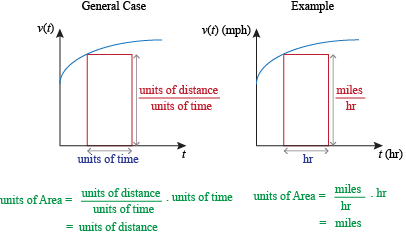We can apply this discussion to other rates of change besides velocity functions. Let f(x) be a continuous function with x measured in meters and f measured in wombats. Then the rate of change f '(x) is measured in wombats per meter. When we find a left-hand sum for f '(x), the height of each rectangle is measured in wombats per meter and the width of each rectangle is measured in meters. This means the area of each rectangle should be measured in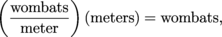which are the units of f. The units stay the same when we add up the areas of many rectangles, and also stay the same when we take the limit as the number of rectangles approaches infinity. This means the units of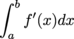are the same as the units of f.

Things get a little weirder when we integrate functions that aren't obviously rates of change. Not harder, just weirder. If f(x) is continuous on [a ,b], we can integrate it, even if f is measured in wombats. Assuming x is still measured in meters, what are the units of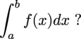Again, we multiply the units of the thing we're integrating (f) by the units of the variable of integration (x). This gives us units of wombat-meters. We don't know what a wombat-meter is, but we know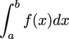is measured in wombat-meters.

The moral of the story is that to find the units of, multiply the units of the integrand by the units of the variable of integration. When we're integrating a rate of change, the units of the independent variable will cancel out and we'll end up with the units of the original function.

When we use an integral to find the average value of a function, the units of the average value are the same as the units of the function. If f(x) is measured in wombats, then the average value of f should also be measured in wombats.

We can analyze the units of the average value in a little more depth. The average value of f on [a, b] is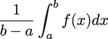which is equivalent to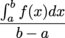We already know the units of the integral are the units of f multiplied by the units of x. The units of (ba) are also the units of x. So the average value is measured in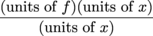The units of x cancel out and we're left with the units of f, as we expected.